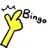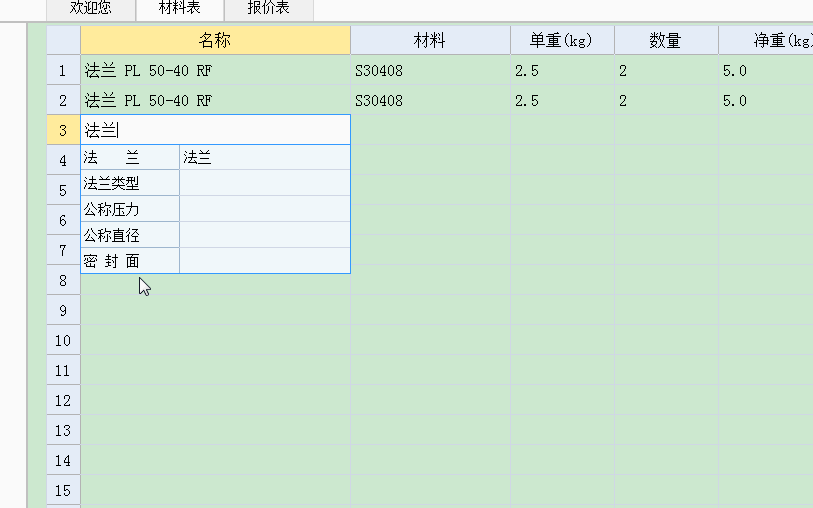# 设备报价_V2.0.1_20191022_测试版### 简要帮助

1. 材料表, 用于统计零部件的重量及价格, 包含多种部件计算模块
2. 报价表, 用于对材料表汇总, 可以按预定模板展示, 并生成标价表单

锥壳、筒体、钢管、圆板、圆环、封头、法兰、支座、方形、型钢、人孔、手孔、弯头、三通、四通

1. 统计公式, 包含“数量、净重、重量、价格, SUM、RMB”
数量: 对材料表中数量一列数字求和
净重: 对材料表中净重一列数字求和
重量: 对材料表中重量一列数字求和
价格: 对材料表中价格一列数字求和
SUM: 对报价表中指定区域求和
RMB: 将报价表中数字转为大写

举例:
重量("Q345R")　　　　　　　　统计一种材料
重量("Q345R" + "S30408")　 统计多种材料
重量(A1)　　　　　　　　　　 材料名称可以是报价表中的单元格引用
重量(A1+A2)　　　　　　　　　多种引用
重量("S*")　　　　　　　　　 支持通配符
SUM(A1:B5)　　　　　　　　　报价表中区域统计
RMB(123)　　　　　　　　　　直接转换
RMB(A1)　　　　　　　　　　 引用转换
RMB(A1+A2)　　　　　　　　　多种引用转换

2. 数学计算
可以进行算术和函数计算, 参照excel
可用函数：PI(), sin(n), cos(n), tan(n), sqrt(n), asin(n), acos(n), atan(n),
lg(n), ln(n), exp(n), abs(n), ceil(n), floor(n)

3. 其他使用习惯可以参照 Excel

公司及地址 lib/config.txt
材料及单价 lib/material.txt